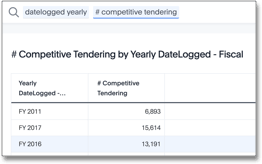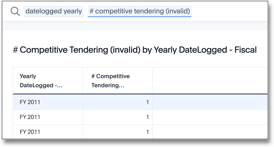# Reaggregation Scenario 1: Supplier tendering by job

We have a fact table at a job or supplier tender response aggregation level. There are many rows for each job, where each row is a single row from a supplier. A competitive tender is a situation when multiple suppliers bid on the same job.

Our objective is to determine what percentage of jobs had more than 1 supplier response. We want to see high numbers, which indicate that many suppliers bid on the job, so we can select the best response.

## Valid solution

A valid query that meets our objective may look something like this:

```sum(group_aggregate(if(sum(# trades tendered ) > 1) then 1 else 0,
query_groups() + {claimid, packageid},
query_filters()))```## Resolution

1. The `sum ( # trades tendered )` function aggregates to these attributes:

• `{claimid, packageid}`

The job-level identifier

• `query_groups( )`

Adds any additional columns in the search to this aggregation. Here, this is the `datelogged` column at the yearly level.

• `query_filters ( )`

Applies any filters entered in the search. Here, there are no filters.

2. For each row in this virtual table, the conditional `if() then else` function applies. So, if the sum of tendered responses is greater than 1, then the result returns 1, or else it returns 0.

3. The outer function, `sum()`, reaggregates the final output as a single row for each `datelogged` yearly value.

• This reaggregation is possible because the conditional statement is inside the `group_aggregate` function.

• Rather than return a row for each `{claimid,packageid}`, the function returns a single row for `datelogged yearly`.

• The default aggregation setting does not reaggregate the result set.

## Non-Aggregated Result

We include the following result to provide contrast to an example where ThoughtSpot does not reaggregate the result set. Reaggregation requires the aggregate function, `sum`, to precede the `group_aggregate` function.

In the following scenario, the next statement is the conditional `if` clause. Because of this, the overall expression does not reaggregate. The returned result is a row for each `{claimid,packageid}`.

```sum(if(group_aggregate (sum (# trades tendered),
query_groups() + {claimid, packageid},
query_filters ( ) )>1) then 1 else 0)```